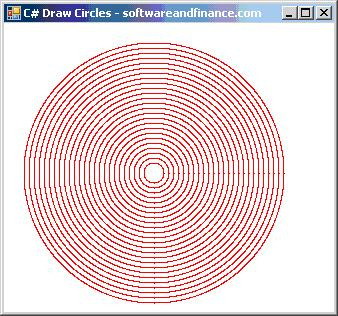﻿ C# - Drawing Circles using Pixel by Pixel

# C# - Drawing Circles using Pixel by Pixel

Drawing Circle is not difficult by using built in function. But if you would like to draw circle pixel by pixel by calculating each cordinates, you can use the formula x + r cos(a) and y + r sin (a).

## Source Code

void PutPixel(Graphics g, int x, int y, Color c)

{

Bitmap bm = new Bitmap(1, 1);

bm.SetPixel(0, 0, Color.Red);

g.DrawImageUnscaled(bm, x, y);

}

private void Form1_Paint(object sender, PaintEventArgs e)

{

Graphics myGraphics = e.Graphics;

myGraphics.Clear(Color.White);

for (int j = 1; j <= 25; j++)

{

radius = (j + 1) * 5;

for (double i = 0.0; i < 360.0; i += 0.1)

{

double angle = i * System.Math.PI / 180;

int x = (int)(150 + radius * System.Math.Cos(angle));

int y = (int)(150 + radius * System.Math.Sin(angle));

PutPixel(myGraphics, x, y, Color.Red);

}

}

myGraphics.Dispose();

}

## Output